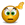# Time difference

## Excel Help for Time Difference in Analyzing Corporate Financial DataDear Sir am waiting for your answer i want do take idle time for machine. so how to calculate the time 11.30 to 13 how to calculate the time in exel sir.    i don't want do mention the date and 12hoursnote: i want use 24hours joneshkumar g Posted by jonus.venus on 31 Mar 2012Rate this: (3/5 from 1 vote) OK - So we assume here that the decimal part of the time represents the number of minutes.  the solution here is to separate the whole number (hours) and the decimal (minutes) to calculate the number of hours difference. We can do this by using the INT function that returns the whole number and then process the decimal part to calculate the fraction of an hour.Assuming that the start time is in cell A1 and the end time is in B1, the formula to calculate the number of hours difference is:=INT(B1-A1)+((B1-A1)-INT(B1-A1)-0.4)/0.6 Excel Business Forums Administrator Posted by Excel Helper on 31 Mar 2012